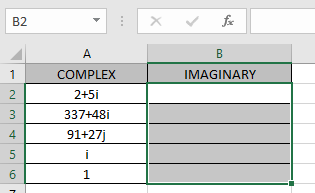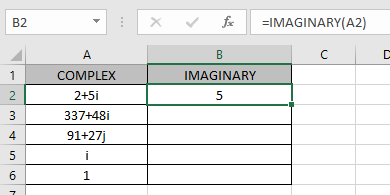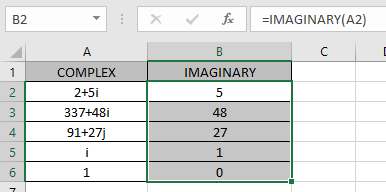# How to use the IMAGINARY Function in Excel

COMPLEX number (inumber) in excel derived for mathematical number having real and imaginary coefficients. In mathematics we call it the coefficient of i or j.

i = (-1)1/2

Square root of negative number is not possible, so for calculation purpose, -1is named as imaginary and call be iota (i or j). For calculation of some term like shown below.

A = 2 + (-25)1/2

A = 2 + (-1 * 25)1/2

A = 2 + (-1 * 5 * 5)1/2

A = 2 + 5 * (-1)1/2

X + iY = 2 + 5i

This here equation is Complex number (inumber) having 2 different parts called real part & complex or imaginary part

The coefficient of iota (i) which is 5 is called as imaginary part and the other part 2 is called the real part.

The IMAGINARY function returns the coefficient of real part  from the argument complex number (inumber) having both real & imaginary part.

Syntax:

=IMAGINARY (inumber)

inumber : complex number having both real & imaginary
Let’s understand this function using it in an example.Here we have values where we need to extract the real part of the complex number (inumber)

Use the formula:

=IMAGINARY (A2)

A2 : complex number (inumber) given argument as cell reference.As you can see the complex number having real_num = 2 & imaginary part = 5. The formula returned the value of real part which is 2 here.

Now copy the formula to the other remaining cells using Ctrl + D shortcut key.As you can see the IMAGINARY function formula giving results just fine.
The table show here explains more about the results

 inmuber Real part Imaginary part = i = 0 + 1i 0 1 = 1 = 1 + 0i 1 0

Note : the formula returns the #NUM! error if the complex number have lower case i or j (iota).

Hope you understood how to use IMAGINARY function and referring cell in Excel. Explore more articles on Excel mathematical functions here. Please feel free to state your query or feedback for the above article.

Related Articles

How to use the IMEXP Function in Excel

How to use the IMSIN Function in Excel

How to use the IMSUM Function in Excel

How to use the IMSUB Function in Excel

How to use the SQRT Function in Excel

How to use the IMARGUMENT Function in Excel

How to use the IMCOS Function in Excel

Popular Articles:

50 Excel Shortcuts to Increase Your Productivity

How to use the VLOOKUP Function in Excel

How to use the COUNTIF in Excel 2016

Edit a dropdown list

If with conditional formatting

If with wildcards

Vlookup by date

Terms and Conditions of use

The applications/code on this site are distributed as is and without warranties or liability. In no event shall the owner of the copyrights, or the authors of the applications/code be liable for any loss of profit, any problems or any damage resulting from the use or evaluation of the applications/code.